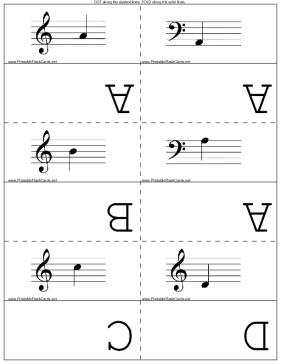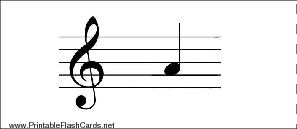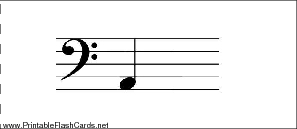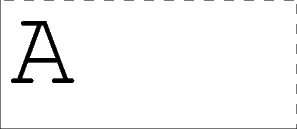Sample flash cards in this set:

Front:Back:This set includes the following cards:
(Image)A
(Image)A
(Image)B
(Image)A
(Image)C
(Image)D
(Image)D
(Image)E
(Image)E
(Image)F
(Image)F
(Image)G
(Image)G
(Image)A
(Image)B
(Image)B
(Image)C
(Image)B
(Image)B
(Image)C
(Image)D
(Image)E
(Image)E
(Image)F
(Image)F
(Image)G
(Image)G
(Image)C
(Image)D
(Image)D
(Image)E